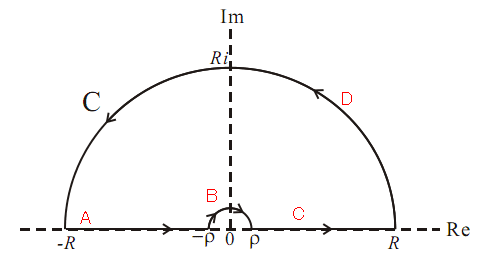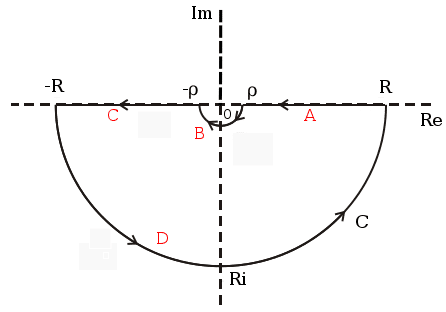## Plotting radial integrated profile from FITS images

Sometimes, you need to know the total power in a particular radius of a FITS image, and the growth of this total power along the radius you choose for checking whether the object is extended or not, or contains some ring or disk structures. Therefore, this script could be very helpful for those kinds of quick checks.

Under the help of Zhangzheng Wen, who gave the idea of calculating the distance from a certain pixel to the image centre, I wrote this IDL radial_power.pro: (Download HERE)

;+ ; NAME: ; RADIAL_POWER ; ; PURPOSE: ; Calculating the radial_power, returning radius and normalized ; integrated power within the radius. ; ; CALLING SEQUENCE: ; PRO radial_power, image, radius, integrated ; ; INPUTS: ; image: read image from a FITS file and put it into a variable. ; ; KEYWORD INPUTS: ; ... ; ; KEYWORDS SWITCHES: ; ... ; OUTPUTS: ; radius: the radius from center to each circle. ; integrated: the normalized integrated power within the radius. ; ; EXAMPLES: ; IDL&gt; image =... ; ;input the image into the variable after read the image from FITS file or other file format. ; IDL&gt; radial_power, image, radius, integrated ; ;this will return the radius, and integrated. ; ; SIDEEFFECT: ; ... ; ; RESTRICTIONS: ; This pro is only useful for one peak structure, namely if ; you want to study two objects with comparable peak flux, ; this pro is not available, but you can edit it to make it ; function well. ; NEED "wherenan.pro" ; ; MODIFICATION HISTORY: ; Write, Chentao YANG (yangcht@gmail.com), 2013-02-04 ; ;-   PRO radial_power, image, radius, integrated   ; make NaN values = 0 IF (SIZE(WHERE(FINITE(image,/NAN)), /N_ELEMENTS) GT 1) THEN image[WHERE(FINITE(image,/NAN))]=0   ; get the index of the maximum point s = SIZE(image,/dimensions) m = (WHERE(image EQ MAX(image))) y_max = m/s x_max = m MOD s   image_size = SIZE(image) image_xdist = FINDGEN(image_size)#TRANSPOSE(FINDGEN(image_size)*0+1) image_ydist = TRANSPOSE(FINDGEN(image_size)#TRANSPOSE(FINDGEN(image_size)*0+1)) image_xdist = (image_xdist-x_max)^2 image_ydist = (image_ydist-y_max)^2 image_dist = SQRT(image_xdist+image_ydist)   radius = FINDGEN(SQRT(MIN([MAX(image_xdist), MAX(image_ydist)]))*0.7) integrated = radius FOR i=0, (SIZE(radius, /N_ELEMENTS)-1) DO BEGIN integrated[i] = TOTAL(image[WHERE(image_dist LE radius[i])]) ENDFOR integrated = integrated/MAX(integrated)   END

## Fourier Transform of a Sinc function

A few days ago, I was trying to do the convolution between a Sinc function and a Gaussian function. But I got stuck from the first step, when I tried to solve that by using the convolution theorem, namely the Fourier transform of the Sinc(x), although I knew it is very easy to find the right answer by Googling or Mathematica. But it worth a try to be done by hand. So, here we go:

We know that the Fourier transform of Sinc(z) is,andSo,

(1)Let us consider the first item, when, namely, we can choose the path below to do the contour integration.Using the method of complex residues, we take the contour with no singular point, separating the path into four parts, namely A, B, C and D shown as the red letters in the figure. Thus,

(2)Also if, namely, and whenand, it is easy to prove using Jordan’s lemma, that the fourth term is equal to zero. Thus we can the rewrite the rest items as,In order to calculate the value of the item on the right side, I use, so, then we have,So, in the end, we get the value of the first integration item shown in the Eq.(1),

(3)When, according to Jordan’s lemma, we can not get the fourth item in the Eq.(2) to be zero. So we have to choose another path for finalising the integration.
So I chose the path below to deal with such a situation,It is not hard to calculate the same way as the previous one,

(4)and here again I use the same trick,, so. Because,So, in the end,

(5)Now, let’s move on to the second part in the Eq.(1). Following the same steps, using contour above real axis I get,

(6)and using contour blow real axis I get,

(7)Finally, we can write the answer as, ifand ifand(Note: if we define the Fourier Transform asThen the result could be a little different.)

## Forwarding port behide a router

Yesterday I was trying to configure my computer behind a router to enable it for the SSH connection from outside the router. After some google-ing, I found many ways all involving changing the iptables rules, but all turned out to be failures. Finally, under the help of Daizhong, I found the working way to configure my computer in order to solve the problem.

Before beginning all the operation below, you need to setup the router to fix your IP address and set the forwarding port through the ADMIN page (normally is 192.168.1.1) of your router(two steps, first is fixing your IP address and then set the port forwarding).

The following steps are:
Firstly, you need to edit the SSHD configuration file

vi /etc/ssh/sshd_config

to enable the port forwarding you set on the ADMIN page of your router.
Then, you need to edit file

vi /etc/services

to set the value of ssh port to the number you gave before.
After that, you should set the firewall either using terminal or UI tools to allow the port for ssh. The terminal way is by adding the following line into the file /etc/sys config/iptables:

-A RH-Firewall-1-INPUT -m state --state NEW -m tcp -p tcp --dport XXXX -j ACCEPT

put your port number to the position of “XXXX”.
In the end, you should set the port allowed under SELinux using:

 sudo semanage port -a -t ssh_port_t -p tcp XXXX

In the end, you can restart the sshd and iptables for a test, by using:

service sshd restart service iptables restart ssh username@ip -p XXXX

.

Note all the commands above need superuser account.

## Filling spectral plot (psym=10) in IDL

Two days ago I encountered a problem with plotting spectra using

plot, x, y, PSYM=10

in IDL when I tried to fill the area between the signal and continuum with some color. I firstly tried the POLYFILL, but failed with the PSYM, namely unable to set PSYM=10 if using POLYFILL directly. Then I tried to search if there exists any .pro file written by others for this purpose, but turned out to be nothing. In the end, under the help of Google and Kexin, I found a way through some simple calculation that can solve this by POLYFILL.
Here I attached the .pro file. Hope it can help you as well.

  ;+ ; NAME: ; FILLSPEC ; ; PURPOSE: ; Two days ago I encountered a problem with plotting spectra using: ; plot, x, y, PSYM=10 ; in IDL when I tried to fill the area between the signal and continuum ; with some color. I firstly tried the POLYFILL, but failed with the PSYM, ; namely unable to set PSYM=10 if using POLYFILL directly. Then I tried to ; search if there exists any .pro file written by others for this purpose, ; but turned out to be nothing. In the end, under the help of Google and ; Kexin, I found a way through some simple calculation that can solve this ; by POLYFILL. ; ; CALLING SEQUENCE: ; fillspec, x, y, continuum, psym=, linecolor=, fillcolor= ; ; INPUTS: ; x, y, continuum. The velocity/frequency/wavelength, flux value and ; the continuum value. ; ; KEYWORD INPUTS: ; psym =: Set the plotpsym. For plotting the spectrum, we use 10. ; linecolor =: Set the spetrum stroke color. ; fillcolor =: Set the color that fill the space between flux value ; and the continuum value. ; ; KEYWORDS SWITCHES: ; ... ; ; OUTPUTS: ; ... ; ; EXAMPLES: ; IDL> x = ... ; input the velocity/frequency/wavelength array ; IDL> y = ... ; input the corresponding flux value array ; IDL> continuum = ... ; input the corresponding continuum value (array) ; IDL> fillspec, x, y, continuum, psym=10, linecolor=220, fillcolor=50 ; ; SIDEEFFECT: ; ... ; ; RESTRICTIONS: ; (Please check if all the variables are in the same order) ; ; MODIFICATION HISTORY: ; Write, 2012-04-21, Chentao Yang (yangcht@gmail.com) ; Only for filling the spectrum after plot the spectrum using ; plot and set PSYM as 10. ; ; Edited on 2015-01-20, Chentao Yang, ; Fix the bug when values beyong YRANGEs are presented. ; ; Edited on 2015-07-17, Chentao Yang, ; Fix the bug when the continuum is not a constant value. ; Fix the bug that this pro may change the variables. ;-     PRO fillspec, x0, y0, cont0, LINECOLOR=LINECOLOR, psym=psym, FILLCOLOR=FILLCOLOR loadct=5   x_sort=sort(x0) x=x0(x_sort) y=y0(x_sort) n=n_elements(x)   OPLOT, x, y, COLOR=LINECOLOR, psym=psym   ; Calculate the additional x-values xnew = (x(0:n-2) + x(1:n-1)) / 2.0D   ; Create array containing all x-values xx = REBIN(xnew, N_ELEMENTS(xnew)*2, /sample) xx = [min(x), min(x), xx, max(x),max(x)]   ; Create array with the corresponding y-values yy = REBIN(y, N_ELEMENTS(y)*2, /Sample)   ; Check if the continuum is a constant IF (N_ELEMENTS(cont0) NE 1) THEN BEGIN cont=cont0(x_sort) yy = [cont, yy, cont[n_elements(cont)-1]] ENDIF ELSE BEGIN yy = [cont0, yy, cont0] ENDELSE   y_mi_indx = where(yy LT !Y.CRANGE) y_ma_indx = where(yy GT !Y.CRANGE) IF (y_mi_indx GT 0) THEN yy[y_mi_indx]=FLTARR(N_ELEMENTS(y_mi_indx))+!Y.CRANGE IF (y_ma_indx GT 0) THEN yy[y_ma_indx]=FLTARR(N_ELEMENTS(y_ma_indx))+!Y.CRANGE       ; Fill the histogram plot POLYFILL, xx, yy, COLOR=FILLCOLOR   ; Histogram plot OPLOT, x, y, COLOR=LINECOLOR, psym=psym   END